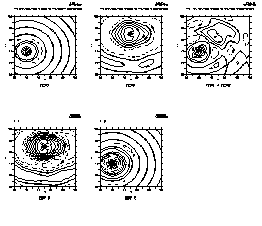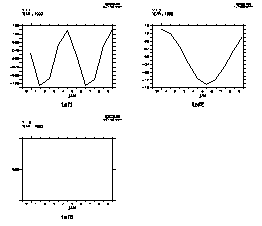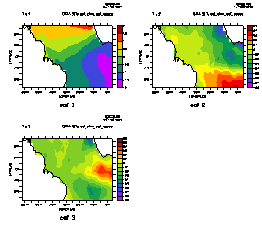How to use Ferret External Functions for computing EOF's

Below is an annotated version of the script ef_eof_demo.jnl

! ef_eof_demo.jnl (8/2000)

```! Description: Demonstration of computing EOFs using the
! External Functions EOF_SPACE, EOF_TFUNC, EOF_STAT```

The functions implements Chelton's '82 method for finding EOFs of gappy time series. All the functions perform the same computation but return different portions of the results. They take time to run as the computation inverts a matrix of size [NT by (NX*NY)]

• EOF_SPACE returns EOF eigenvectors: spatial EOF in x and y with the same units as the incoming data
• EOF_TFUNC returns EOF time amplitude functions; dimensionless
• EOF_STAT returns statistics on the EOF computation: number of EOFs scaled and returned; Percent variance explained by each EOF; and the eigenvalues.

For all functions the arguments are as follows:

• 1st argument: Input data field, a function of x, y, and time; may be a function of z
• 2nd argument: The minimum percent variance explained by the EOF's that are computed and scaled, e.g. to return EOFs that explain at least 2% variance, use 2.0
• 3nd argument: The fraction of each time series that must be present to include it in the calculations, e.g. to use all time series that have at least half the data present, use 0.5
```!
! EOF functions Example 1: Define a function of x,y,time using trig
! functions.  Decompose into spatial and time EOF's and display statistics.
!
yes? LET time = t[t=1-jan-1990:10-jan-1990:24]   ! 24 hour resolution time axis

yes? DEFINE AXIS/x=0:10:0.5 x10
yes? DEFINE AXIS/y=0:10:0.5 y10
yes? DEFINE GRID/x=x10/y=y10/t=time g10x10
yes? SET GRID g10x10

yes? LET fcn1 = 15.* sin(omega1*t)*cos(r)/(r+1)
yes? LET fcn2 = 20.* sin(omega2*t)*(sin(s)-.2*sin(q))/(s+1)

yes? LET r = ((6.*(xpts-x0)^2 + 7.*(ypts-y0)^2)^0.5)
yes? LET s = (((xpts-x1)^2 + 2*(ypts-y1)^2)^0.5)
yes? LET q = ((3*(xpts-x0)^2 + (ypts-y1)^2)^0.5)

yes? LET x0 = 2
yes? LET y0 = 4
yes? LET x1 = 5
yes? LET y1 = 7

yes? LET omega1 = 1/10*2*3.14159
yes? LET omega2 = 2 * omega1

yes? LET sample_function = fcn1 + fcn2
yes? LET xpts = x
yes? LET ypts = y

!  Compute the statistics on the EOF solution.  EOF_STAT returns:
!  for J=1  the number of EOFs returned
!  for J=2  the percent variance explained by each EOF
!  for J=3  the eigenvalue for each EOF

yes? LET estat = eof_stat(sample_function, 0.1)

yes? LIST/I=1/J=1 estat
EOF_STAT(SAMPLE_FUNCTION, 0.1)
X: 1
Y: 1
2.000

yes? LIST/I=1:4/J=2 estat
EOF_STAT(SAMPLE_FUNCTION, 0.1)
2
1   / 1:  93.59
2   / 2:   6.41
3   / 3:   0.00
4   / 4:   0.00

yes? LIST/I=1:4/J=3 estat
EOF_STAT(SAMPLE_FUNCTION, 0.1)
3
1   / 1:  1104.
2   / 2:    76.
3   / 3:     0.
4   / 4:     0.

!  Plot the original function (averaged over time) and its spatial
!  decomposition by EOF_SPACE

yes? DEFINE VIEW/xlim=0.,.33/ylim=.6,1./text=0.2 vul
yes? DEFINE VIEW/xlim=.33,.66/ylim=.6,1./text=0.2 vuc
yes? DEFINE VIEW/xlim=.66,1./ylim=.6,1./text=0.2 vur

yes? DEFINE VIEW/xlim=0.,.33/ylim=.1,.5/text=0.2 vll
yes? DEFINE VIEW/xlim=.33,.66/ylim=.1,.5/text=0.2 vlc
yes? DEFINE VIEW/xlim=.66,1./ylim=.1,.5/text=0.2 vlr

yes? SET VIEW vul; CONTOUR/TITLE="FCN1" fcn1[l=1:10@ave]
yes? SET VIEW vuc; CONTOUR/TITLE="FCN2" fcn2[l=1:10@ave]
yes? SET VIEW vur; CONTOUR/TITLE="FCN1 + FCN2" sample_function[l=1:10@ave]

yes? LET exy = eof_space(sample_function, 0.1)

yes? SET VIEW vll; CONTOUR/L=1/TITLE="EOF 1" exy
yes? SET VIEW vlc; CONTOUR/L=2/TITLE="EOF 2" exyyes? CANCEL VIEW

! Now plot the time amplitude functions.

yes? LET etim = eof_tfunc(sample_function, 0.1)

yes? SET VIEW ul
yes? PLOT/I=1/TITLE=taf1 etim

yes? SET VIEW ur
yes? PLOT/I=2/TITLE=taf2 etim

! should be all bad flags...
yes? SET VIEW ll
yes? PLOT/I=3/TITLE=taf3 etimyes? CANCEL DATA/ALL
yes? CANCEL VARIABLE/ALL
yes? CANCEL REGION

!  A second example, using the COADS climatology data.
!

yes? SET REGION/X=67w:1w/Y=11S:11N

!  Compute and save the spatial EOF functions.  These have the same units
!  as the data.

yes? LET eof_xyfcn = eof_space(sst, 0.5)
yes? SAVE/CLOBBER/FILE=sst_clim_eof_space.cdf eof_xyfcn
yes? LISTing to file sst_clim_eof_space.cdf

yes? CANCEL DATA/ALL
yes? CANCEL VARIABLE/ALL
yes? CANCEL REGION

yes? USE sst_clim_eof_space.cdf
yes? SET VIEW ul; fill/l=1/TITLE="eof 1" eof_xyfcn; go land
yes? SET VIEW ur; fill/l=2/TITLE="eof 2" eof_xyfcn; go land
yes? SET VIEW ll; fill/l=3/TITLE="eof 3" eof_xyfcn; go landyes? CANCEL DATA/ALL
yes? CANCEL VARIABLE/ALL
yes? CANCEL REGION

!  Compute the statistics on the EOFs: number of EOFs scaled
!  and returned; Percent variance explained by each EOF; and the
!  eigenvalues.

yes? SET REGION/X=67w:1w/Y=11S:11N
yes? LET eofstat = eof_stat(sst[X=67w:1w,Y=11S:11N], 0.5)

yes? let nout = eofstat[i=1,j=1]
yes? let pcts = eofstat[i=1:`nout`,j=2]
!-> DEFINE VARIABLE pcts = eofstat[i=1:3,j=2]
yes? let eigenv = eofstat[i=1:`nout`,j=3]
!-> DEFINE VARIABLE eigenv = eofstat[i=1:3,j=3]

yes? LIST nout
VARIABLE : EOFSTAT[I=1,J=1]
DATA SET : COADS Monthly Climatology (1946-1989)
FILEPATH : /home/ja9/tmap/fer_dsets/descr/
X        : 1
Y        : 1
285.0

yes? LIST/I=1:6 pcts
VARIABLE : EOFSTAT[I=1:285,J=2]
DATA SET : COADS Monthly Climatology (1946-1989)
FILEPATH : /home/ja9/tmap/fer_dsets/descr/
SUBSET   : 6 points (X)
Y        : 2
2
2
1    /  1:  86.62
2    /  2:   5.97
3    /  3:   3.89
4    /  4:   1.53
5    /  5:   0.67
6    /  6:   0.38

yes? LIST/I=1:6 eigenv
VARIABLE : EOFSTAT[I=1:285,J=3]
DATA SET : COADS Monthly Climatology (1946-1989)
FILEPATH : /home/ja9/tmap/fer_dsets/descr/
SUBSET   : 6 points (X)
Y        : 3
3
3
1    /  1:  249.4
2    /  2:   17.2
3    /  3:   11.2
4    /  4:    4.4
5    /  5:    1.9
6    /  6:    1.1

yes? CANCEL REGION

!  Compute and save time amplitude functions: Note they are dimensionless.

yes? LET eoftime = eof_tfunc(sst[X=67w:1w,Y=11S:11N], 0.5)

SAVE/CLOBBER/FILE=sst_clim_eof_tfunc.cdf eoftime[i=1:`nout`]
!-> LIST/FORMAT=CDF/CLOBBER/FILE=sst_clim_eof_tfunc.cdf eoftime[i=1:3]
LISTing to file sst_clim_eof_tfunc.cdf

yes? CANCEL VARIABLE/ALL
yes? CANCEL VIEW
yes? USE sst_clim_eof_tfunc.cdf

yes? SET VIEW ul
yes? PLOT/I=1/TITLE="time function 1"/VLIMITS=-2:2:0.5 eoftime
yes? SET VIEW ur
yes? PLOT/I=2/TITLE="time function 2"/VLIMITS=-2:2:0.5 eoftime
yes? SET VIEW ll
yes? PLOT/I=3/TITLE="time function 3"/VLIMITS=-2:2:0.5 eoftime```

oar.pmel.contact_ferret@noaa.gov 30 Apr, 1999